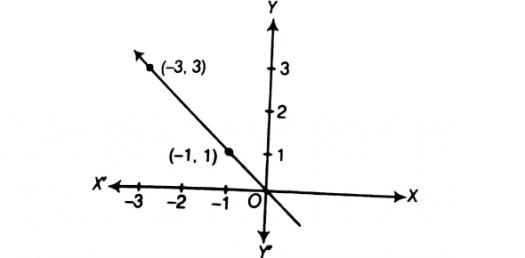# Can You Take The Linear Equation Quiz To Pass The Test?

5 Questions | Total Attempts: 107SettingsCan you pass the linear expression quiz to pass the test? Did you know that in mathematics, a linear equation is one that may be put in the form where the variables are unknown and are the coefficients that are often real numbers? Are you able to understand critical mathematical concepts enough to pass a test? Take this quiz and find out!

• 1.
Which equation correctly shows this relationship? xy-1 -50-211
• A.

Y = -3x - 2

• B.

Y = 3x - 2

• C.

Y = 1/3x + 2

• D.

Y = -2x + 3

• 2.
What is the y-intercept in this situation?xy-2-12-4-8
• A.

- 16

• B.

8

• C.

- 8

• D.

- 12

• 3.
Which equation does NOT match this situation?Lin is tracking the growth of her plant in science class. Today it is 5 cm tall and it grows 1.5 cm per day.
• A.

Y = 5x + 1.5

• B.

Y = 5 + 1.5x

• C.

1.5x + 5 = y

• 4.
Kara used the linear model y = 20,000 + 0.3x to predict her total salary if she has a sales amount of x. What is her base salary?
• A.

20,000

• B.

0.3

• C.

60,000

• D.

You cannot tell from this information.

• 5.
Kara used the linear model y = 20,000 + 0.3x to predict her total salary if she has a sales amount of x. What percent commission does she earn??
• A.

30%

• B.

0.3

• C.

20,000

• D.

You cannot tell from this information.

Related TopicsBack to top